##### Search### SECTION I (50 Marks)

Answer all the questions in this section in the spaces
1.

Find using logarithms , the value of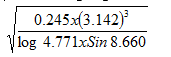3 marks

2.

. Make the letter Z the subject of the formula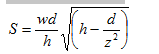3 marks

3.

In finding the area of a rectangle whose dimensions are 7.28 by 5.49, a student truncated the measurement to 1d.p. Find the percentage error arising from this.

2 marks

4.

Simplify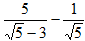leaving your answer in the form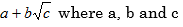are rational numbers.

3 marks

5.

. The cost per month of running a private school is partly constant and partly varies directly with the number of pupils. If it cost sh.300000 a month when there are 400 pupils and sh. 420,000 a month when there are one and a half time that number. Find the cost of running a school of 1000 pupils.

3 marks

6.

a) Expand and simplify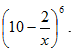2 marks

7.

The difference between the first two terms of a geometric progression is 16. While the difference between the second and third terms is 64. Find the sum of the first 12 terms.

3 marks

8.

Solve the simultaneous equations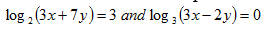2 marks

9.

A line segment joining two points P(0 ,7) and S(2, 3.8) is divided externally by point Q in the ratio 7 :3. Find the co-ordinates of point Q.

3 marks

10.

The figure below shows a square ABCD of length 4cm. M and N are the midpoints of opposite sides of the square ABCD. A point O lies inside the square. Find the probability that it lies in triangle ADM but not in triangle ADN.

3 marks

11.

Solve the equation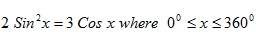3 marks

12.

After a transformation given by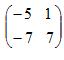the image has an area of 112 square

units. Find the area of the object.

2 marks

13.

. Find the equation of the normal to the curve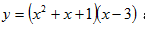at the point where it cuts the x- axis.

3 marks

14.

. Find the length of the tangent from the point ( 5,7) to the circle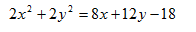3 marks

15.

. A ship leaves an island P (600N, 800E) on 0300Hrs Monday and sails due east for 15 hours to another Island Q. If the average speed of the ship is 46 knots, find the time of arrival of the ship on Island Q.

4 marks

16.

The figure below shows a triangular piece of land ABC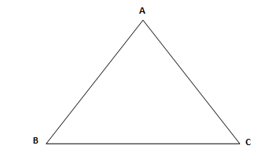Locate the position of T and X given that:-

a) A tap T is installed inside the plot such that it is equidistant from all the three edges. (2mks)

b) A portion of the plot represented by the variable X that is closer to the edge AC than BC and that angle AXB Is less than 900.

3 marks

### SECTION II (50 Marks)

Answer only five questions in this section in the spaces provided.
17.

. In an experiment, L loads were hang on one end of a coiled spring, and the extensions E recorded in the table below.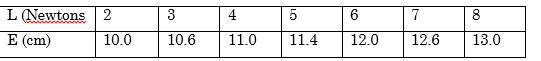a) Using a scale of 1cm to re present one unit on the vertical axis and 2cm to represent one unit on the horizontal axis, draw a suitable straight line graph of extension against the load. (3mks) Grid

b) From the graph, estimate i) The natural length of the spring (1mk

ii) The extension of the spring when the load is 1N. (1mk)

iii) The load that produces an extension of 11.4cm. (1mk)

c) i) Establish the law connecting L and E. (3mks)

ii) Use the law in

(c) i) above to find the extension when the load is 5.5N.

6 marks

18.

60 Governors recorded the number of times they visited their respective counties as follows.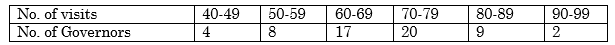Draw a cumulative frequency curve for the data above.

Use your graph to estimate i) Median (1mk)

ii) Quartile deviation (3mks)

iii) The number of Governors who were able to visit counties between 51

5 marks

19.

A water tank is filled by means of two pipes of different diameters. The smaller of the two takes 5 minutes longer to fill the tank than the larger one. If both pipes are used at the same time, it takes 6 minutes to fill the tank. a) How long would it take each pipe to fill the tank? (5mks)

b) If the two pipes are opened together for two minutes then the larger one is closed, how much longer does it take the smaller pipe to fill the tank? (2mks)
c) If the tank is to be fed by the larger tap only which is of diameter 4.2cm, the water flows at the rate of 5cm/s, find the volume of the tank in litres.

7 marks

20.

. In the figure below A, B, C and D are points on the circumference of a circle centre O.

The points E, D, O and B lie on a straight line. FAE is a tangent to the circle at A. <BOC=1100 and <ABE=300.Find the value of the following angles, stating the reasons in each case.

a) <DCA (2mks)

b) <OCA (2mks)

c) <CAE (2mks)

d) <DAE (2mks)

e) < BAF (2mks)

3 marks

21.

. a) The cash price of a 30 inch plasma TV is Ksh.30,000. A lady bought it on hire purchase by paying 10% of the cash price as deposit and 24 monthly instalments. If the hire purchase price is 1 ½ times the cash price, calculate

i) the size of each instalment. (2mks)

ii) the rate of interest per annum. (2mks)

b) A ranch 20km from Rumuruti township was valued at fourty five million shillings at the end of 2005. Due to insecurity the ranch depreciated in value by 20% in 2006, 15% in 2007, 10% p.a, in 2008 and 2009 respectively. Since 2010 after the enactment of the new constitution and Rumuruti township was gazetted as the new county headquarters of Laikipia County, the value of the ranch has been appreciating uniformly at the rate of 10% p.a.
i) What was the value of the ranch at the end of 2009? (4mks)

ii) What will be the value of the ranch at end of the year 2013? (2mks)

8 marks

22.

. The figure below represents a prism with a trapezoidal cross-section. AB =5m, BG=3m, BC=8m and CF=9m.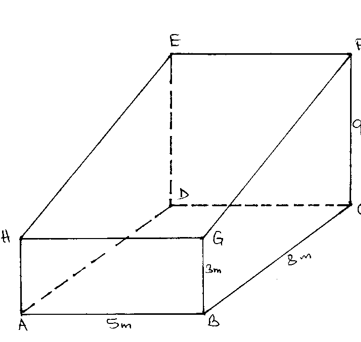Calculate a) The length of AF (2mks)

b) The angle between AF and the plane ABCD. (2mks)

c) The angle between the planes EFCD and EFGH. (2mks)

d) The angle between the planes ABCD and EFGH (2mks)

e) The angle between the plans ABCD and HGCD (2mks)

10 marks

23.

The 4 NTE transport company operates commuter services to the national parks in Kenya. It has two types of vehicles; the shuttle type which us e 120 litres of fuel every day, and the minibus type which uses 180 litres of fuel everyday. The fuel available per month is at least 12000 litres. The company is allowed at least 60 days per year to the national parks. To keep the vehicles in good condition the shuttle must travel for x days per year while the minibus must travel for y days per year. a) Write down all the inequalities representing the above information. (3mks)

b) On the grid provided, draw all the inequalities in

(a) above and shade the unwanted region. (4mks)

Grid

c) The profit on the shuttle is Ksh.3000 per day while that on the minibus is Ksh. 4000 per day. Use the graph in

(b) above to determine the maximum profit that the company makes per year. (3mks)

10 marks

24.

The diagram below shows the graph of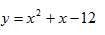a) Determine the co-ordinates of points A and B. (2mks)

b) i) Calculate the area bounded by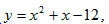, the x-axis and the lines x=0 and x=4 using the mid-ordinate rule with 4 ordinates. (3mks)

ii)Find the exact area

ii)Find the exact area in (b)

(i) above (3mks)

c) Hence calculate the percentage error in using the mid-ordinate rule. (2mks)

10 marks

Back Top